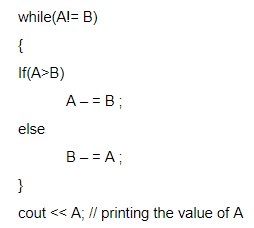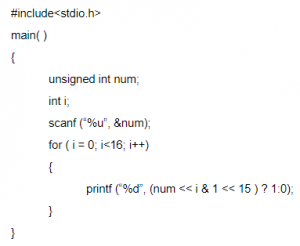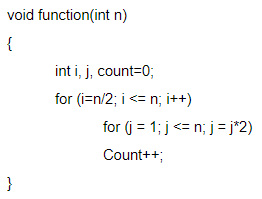## Control Flow

 Question 1
Assume A and B are non zero positive integers. The following code segmentA Computes the LCM of two numbers B Divides the larger number by the smaller number C Computes the GCD of two numbers D Finds the smaller of two numbers
Programming-for-Output-Problems       Control Flow       ISRO-2018       Video-Explanation
Question 1 Explanation:
→ The above iterative code is computes the GCD of two numbers using euclidean algorithm.
→ The procedure is to subtract smaller number from larger. So that we can reduce larger number then doesn’t change the value of GCD.
→ if we performing number of iterations based on condition the larger of two numbers will end up with GCD.
 Question 2
Consider the following C programHow many lines of output does this program produce?
 A 0-9 lines of output B 10-19 lines of output C 20-29 lines of output D More than 29 lines of output
Programming-for-Output-Problems       Control Flow       ISRO-2018       Video-Explanation
Question 2 Explanation:
→ Since there is one line of output for each loop, we need to determine the number of
times the loop executes. Since i is constant, we need to see the growth of j only.
→ Let the initial value of j be denoted by J1 and the subsequent values by Jn for n= 2, 3,... so that J denotes a progression of j. We see that Jn = 2Jn-1;
→ J1 = 2, which gives Jn = 2n-1;
n = 1, 2, ...
→ The loop will execute as long as i/j=2.0/2n-1 >0.001 which gives n<3log210+2 (or) n<11.97.
→ Thus the loop will execute 11 times which is equivalent to say there will be 11 lines of output.
 Question 3
What will be the output of the following C code?A 10 11 12 13 14 B 10 10 10 10 10 C 0 1 2 3 4 D Compilation error
Programming       Control Flow       ISRO-2017 May       Video-Explanation
Question 3 Explanation:
Step-1: We are initialized i=0 in for loop. It means condition true because it is less than 5.
Step-2: Inside the for loop we are assigning again I value is 10 and printing value i.
Iteration-1: We are printing value 10 then increment by 1
Iteration-2: We are clearing previous value and assigning value 10. Printing value is 10;
Iteration-5: We are clearing previous value and assigning value 10. Printing value is 10
Step-3: output is 1010101010
 Question 4
What does the following program do when the input is unsigned 16-bit integer?A It prints all even bits from num B It prints all odd bits from num C It prints binary equivalent of num D None of the above
Programming       Control Flow       ISRO-2017 May       Video-Explanation
Question 4 Explanation:
Step-1: Take n value initially 14(any number we can take but we are taken 14)
Step-2: 14<16
Step-3: It will print 00000000 00001110
 Question 5The complexity of the program is
 A O(log n) B O(n2) C O(n2 log n) D O(n log n)
Programming       Control Flow       ISRO-2017 December       Video-Explanation
Question 5 Explanation:There are 5 questions to complete.

Register Now